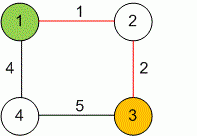Competitions

# The Shortest Path

The undirected weighted graph is given. Find the shortest path between two given vertices.

#### Input

The first line contains two positive integers n and m (n2000, m50000) - the number of vertices and edges in a graph. The second line contains positive integers s and f (1s, fn, sf) - the numbers of the vertices, the minimal length between which must be found. Each of the next m lines contains three numbers bi, ei and wi - the numbers of the ends of i-th edge and its weight correspondingly (1bi, ein, 0wi100000).

#### Output

Print in the first line one number - the length of minimal path between the vertices s and f. Print in the second line all the vertices on the shortest path from s to f in the traversal order. If the path from s to f does not exist, print -1.Time limit 2 seconds
Memory limit 128 MiB
Input example #1
4 4
1 3
1 2 1
2 3 2
3 4 5
4 1 4

Output example #1
3
1 2 3